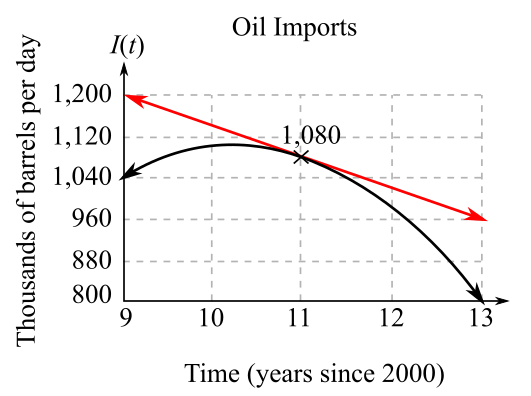Chapter 10.5, Problem 81EFinite Mathematics and Applied Cal...

7th Edition
Stefan Waner + 1 other
ISBN: 9781337274203

Solutions

Chapter
SectionFinite Mathematics and Applied Cal...

7th Edition
Stefan Waner + 1 other
ISBN: 9781337274203
Textbook Problem

Oil Imports from Mexico The following graph shows approximate daily oil imports to the United States from Mexico.60 Also shown is the tangent line at the point corresponding to year 2011.a. Estimate the slope of the tangent line shown on the graph. What does the graph tell you about oil imports from Mexico in 2011? [HINT: Identify two points on the tangent line. Then see Quick Example 3.]b. According to the graph, is the rate of change of oil imports from Mexico increasing, decreasing, or increasing then decreasing? Why?

(a)

To determine

The slope of the tangent of the line passing through the year 2011 and its interpretation by the graph showing daily import of oil for U.S. from México vs. the year (since 2000

).Explanation

Given information:

The graph of daily import of oil for U.S. from México vs. the year (since 2000) is:

From the graph, the tangent line passes through the two points, first point is highlighted as (11,1080) and the second point is taken by the end of tangent line, that is, (13,960).

The slope by using the two points (11,1080) and (13,960) is as follows:

m=y2y1</

(b)

To determine

If the rate of change of oil import from Mexico vs. year is increasing or decreasing. The graph of daily import of oil for U.S. from México vs. the year (since 2000) is:Still sussing out bartleby?

Check out a sample textbook solution.

See a sample solution

The Solution to Your Study Problems

Bartleby provides explanations to thousands of textbook problems written by our experts, many with advanced degrees!

Get Started

In Exercises 3-11, find the derivative of the function. f(x)=12x

Calculus: An Applied Approach (MindTap Course List)

Solve the equation for x. 46. |2x1x+1|=3

Single Variable Calculus: Early Transcendentals, Volume I

Let h be the function defined by h(x) = x3 x2 + x + 1. Find h(5), h(0), h(a), and h(a).

Applied Calculus for the Managerial, Life, and Social Sciences: A Brief Approach

In Problem 45-51, solve each system of equations. 45.

Mathematical Applications for the Management, Life, and Social Sciences

The graph of x = cos t, y = sin2 t is:

Study Guide for Stewart's Multivariable Calculus, 8th

For f(x) = x3 + 7x, df = _____. a) (x3 + 7x) dx b) (3x2 + 7) dx c) 3x2 + 7 d) x3 + 7x + dx

Study Guide for Stewart's Single Variable Calculus: Early Transcendentals, 8th